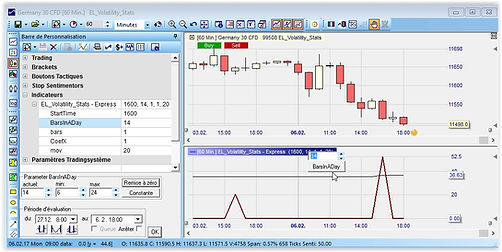top of page#### Volatility Stats Indicator

Which hour is the best for big trend starts for DAX ?

How much volatility can I expect at this hour ?

How much volatility is already consumed?

Is this stocks index able to give me enough volatility for profitable trading ? And when ?

You can answer all this questions and more with this simple but powerfull indicator.###### EL_Volatility_Stats-Express

is the name of this express indicator. EL is for Eric Lefort, like a lot of Mogalef indicators. You must put this text file in your Nanotrader Express directory.

On this one hour DAX example, you can see that the 20 days average volatility between 16h and 17h is 51.86 points. ( at this date)###### CAC DAX DOW volatility (1 hour) on 2017/02/05

EL_Volatility_Stats-express indicator allows to collect the necessary data to make curves as those opposite.

Hour by hour, you can see here the CAC, DAX and DOW one hour volatility. With this graph it's easy to know when it's a good time to trade with a favourable volatility.

Also, according to the hour, it is easy to see on which stock index it is interesting to trade to benefit from a maximum of volatility.

###### 2017/02/05 datas

EL_Volatility_Stats-express indicator allows to collect the necessary data to make curves as those opposite..

Hour by hour, you can see here the EUR/USD, GBP/USD et US Dollar Index one hour volatility. With this graph it's easy to know when it's a good time to trade with a favourable volatility.

Be carefull : To have exploitable values on the FOREX, it is necessary to understand well the use of the parameter COEFX. It is indeed necessary to multiply the values of volatility to be able to exploit them because the FOREX has very small variation (hundredth, thousandth).

#### Indicator's parameters

For an hourly graph, you will need to make vary the parameter Start-Time (and Bars in a Day if necessary)###### START TIME

This is the principal parameter. It allows you to choose at which time slot you want to see volatility and volatility moving average.

Hour is four numbers formating (here 1600).

On this DAX exemple you can see that the 20 days moving average of hour volatility (between 16h and 17h) is 51.86 points.###### Bars In A Day

So that the indicator can give you a correct value of the average of volatility, you should indicate him how many bars are in one day.

For example, the CAC index have 14 hourly bars in a day (from 8h00 to 21h59).

DAX future is 14 hours a day, but DAX CFD is 24 hours a day (Unless configured differently).

EUR/USD is 24 hours a day, but other pairs are only 23. It belongs to you to indicate this parameter to the indicator so as to obtain an exact moving average.###### BARS

Default value is 1 : we examine the volatility on one bar, for example over one hour in hourly graph.

In this example the parameter is 2 and the hour is 1600. The result will be the volatility between 15h and 17h (the 16h present bar and the previous one).

Per example, this parameter is usefull if you want to know (with a 30mn graph) what is the volatility between 15h30 and 16h30.###### CoefX

It is a coefficient multiplier.

Useless for DAX or other index, because we need volatility in points. Nevertheless you can change it if you prefer to have volatility in ticks

Usefull for the FOREX.

For a better legibility, it can be useful to have the value of the volatility in thousandth of points.
For example for the EUR / USD, the parameter is fitted to 1000, or 10000...

CoefX de Volatility Stats###### MOV

Moving average period.

Default value is 20.

Example : 20 days moving average of 16h-17h volatility.